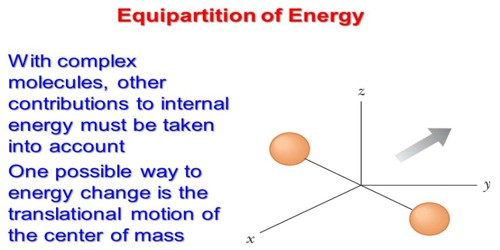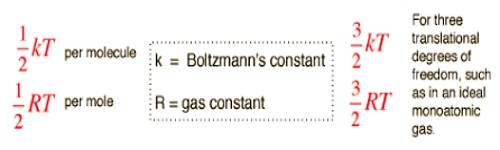# The Law of Equipartition of Energy

The Law of Equipartition of Energy

Before discussing the law of equipartition of energy we need to know what is ‘degrees of freedom’.

Degrees of freedom: Numbers of independent variable quantities that are needed to express the state of motion of a body or position completely are called degrees of freedom.

A monoatomic gas molecule has three degrees of freedom. A diatomic gas molecule has five degrees of freedom- three for linear motion and two for rotational motion.

Equipartition principle of Energy:

The law of equipartition of energy sates that for any dynamical system in a thermal equilibrium, the total energy is equally divided among the degrees of freedom. The energy associated with a molecule for one degree of freedom is ½ KT where k is the Boltzmann’s constant and T is the temperature.The total energy of a thermodynamic system in thermal equilibrium is distributed equally in each degree of freedom and amount of energy in each degree of freedom = ½ KT. According to the law of equipartition of energy, for any dynamic system in thermal equilibrium, the total energy for the system is equally divided among the degree of freedom.

Now we will apply this law to gas molecules. We know, has three degrees of freedom. So, according to this law average energy of a molecule = 3/2 KT. As the total degrees of freedom of diatomic molecules is 5, so the average energy of each molecule = 5/2 KT.

For example, an atom of a gas has three degrees of freedom (the three spatial, or position, coordinates of the atom) and will, therefore, have an average total energy of 3/2 KT. For an atom in a solid, vibratory motion involves potential energy as well as kinetic energy, and both modes will contribute a term ½ KT, resulting in an average total energy of 3 KT.